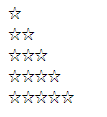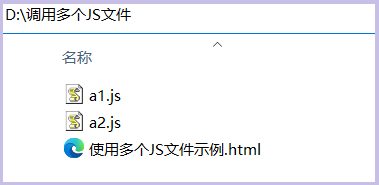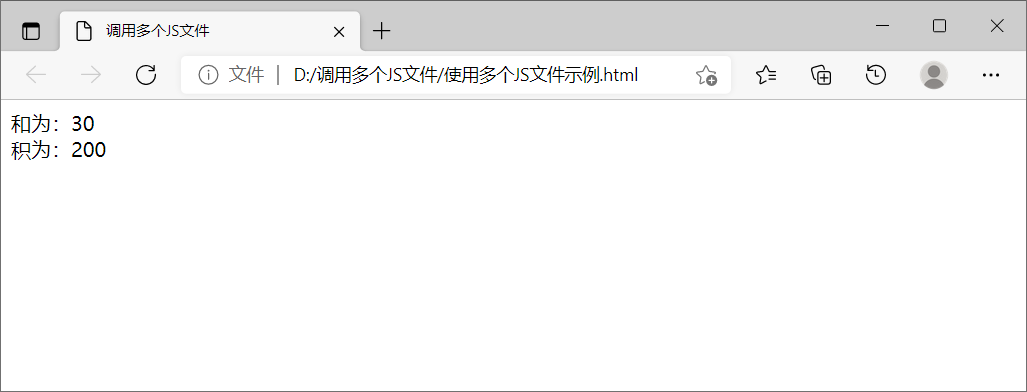### 在HTML文档中使用JavaScript的方法详解function RightTriangle(){

for(var j=1;j<6;j++)

{

for(var i=1;i<=j;i++)

{

document.write("☆");

}

document.write("<br/>");

}

}

1.内嵌，放置在<script>和</script>标签之间，格式如下：

<Script>

JavaScript 语句

</Script>

``````<!DOCTYPE html>
<meta charset="utf-8">
<title>内嵌</title>
<script >
function RightTriangle(){
for(var j=1;j<6;j++)
{
for(var i=1;i<=j;i++)
{
document.write("☆");
}
document.write("<br/>");
}
}

RightTriangle(); //调用函数
</script>
<body>
</body>
</html>

``````

2.放置在有<script>标签的src属性指定的外部文件中，即调用JavaScript文件方法，

<script src = "JavaScript脚本文件"></script>

（1）网页文件【文件名为：用JS生成字符☆直角三角形本（调用JS文件）.html】源码如下：

``````<!DOCTYPE html>
<meta charset="utf-8">
<title>调用JS文件</title>
<script src="TriangleFile.js">
</script>
<script>
RightTriangle(); //调用TriangleFile2.js中的函数
</script>
<body>
</body>
</html>
``````

（2）JavaScript文件【文件名为：TriangleFile.js】源码如下：

``````function RightTriangle(){
for(var j=1;j<6;j++)
{
for(var i=1;i<=j;i++)
{
document.write("☆");
}
document.write("<br/>");
}
}

``````

【特别说明：html页面中允许使用多个js文件，引入的js文件位置和单个文件一样：

<script src=script1.js></script>

<script src=script2.js></script>

</body>

<script src=script1.js></script>

<script src=script2.js></script>

</html>a1.js文件源码如下：

``````function getSum(num1,num2){  //定义求和函数getSum
sum = num1 + num2;
return sum;
}

``````

a2.js文件源码如下：

``````function getMultiply(num1,num2){  //定义求积函数getMultiply
product = num1 * num2;
return product;
}

``````

``````<html>
<title>调用多个JS文件</title>
<script  src = "a1.js"></script>
<script  src = "a2.js"></script>
<script>
var sum = getSum(10,20); //调用a1.js中的getSum()
var Multiply = getMultiply(10,20); //调用a2.js中的getMultiply()
document.write("和为：" + sum);
document.write("<br>");
document.write("积为：" + Multiply);
</script>
<body>
</body>
</html>

``````3.放置自HTML事件处理程序中，该事件处理程序由onclick或onmouseover这样的HTML属性值指定它。HTML事件属性一般以on开头。

<标签名 事件名 = "JavaScript代码或函数"></标签名>

``````<!DOCTYPE html>
<meta charset="utf-8">
<title>在HTML事件中</title>
<script >
for(var j=1;j<6;j++)
{
for(var i=1;i<=j;i++)
{
document.write("☆");
}
document.write("<br/>");
}
}
</script>
<body>
</body>
</html>

``````

4.放在一个URL里，这个URL使用特殊的协议”javascript“协议，这种方式很少使用。

javascript: 要执行的操作

``````<!DOCTYPE html>
<meta charset="utf-8">
<title>在URL中</title>
<script >
function RightTriangle(){
for(var j=1;j<6;j++)
{
for(var i=1;i<=j;i++)
{
document.write("☆");
}
document.write("<br/>");
}
}
</script>